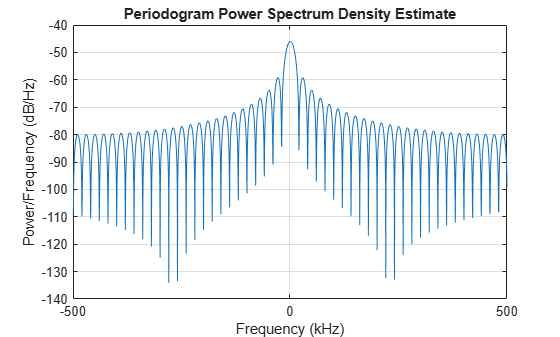getStretchProcessor

System object: phased.LinearFMWaveform
Package: phased

Create stretch processor for waveform

Syntax

HS = getStretchProcessor(H)
HS = getStretchProcessor(H,refrng)
HS = getStretchProcessor(H,refrng,rngspan)
HS = getStretchProcessor(H,refrng,rngspan,v)

Description

HS = getStretchProcessor(H) returns the stretch processor for the waveform, H. HS is set up so the reference range corresponds to 1/4 of the maximum unambiguous range of a pulse. The range span corresponds to 1/10 of the distance traveled by the wave within the pulse width. The propagation speed is the speed of light.

HS = getStretchProcessor(H,refrng) specifies the reference range.

HS = getStretchProcessor(H,refrng,rngspan) specifies the range span. The reference interval is centered at refrng.

HS = getStretchProcessor(H,refrng,rngspan,v) specifies the propagation speed.

Input Arguments

 H Linear FM pulse waveform object. refrng Reference range, in meters, as a positive scalar. Default: 1/4 of the maximum unambiguous range of a pulse rngspan Length of the interval of ranges of interest, in meters, as a positive scalar. The center of the interval is the range value specified in the refrng argument. Default: 1/10 of the distance traveled by the wave within the pulse width v Propagation speed, in meters per second, as a positive scalar. Default: Speed of light

Output Arguments

 HS Stretch processor as a phased.StretchProcessor System object™.

Examples

expand all

Use stretch processing to locate a target at a range of 4950 m.

Simulate the signal.

waveform = phased.LinearFMWaveform;
x = waveform();
c = physconst('LightSpeed');
rng = 4950.0;
num_samples = round(rng/(c/(2*waveform.SampleRate)));
x = circshift(x,num_samples);

Perform stretch processing.

stretchproc = getStretchProcessor(waveform,5000,200,c);
y = stretchproc(x);

Plot the spectrum of the resulting signal.

[Pxx,F] = periodogram(y,[],2048,stretchproc.SampleRate,'centered');
plot(F/1000,10*log10(Pxx))
grid
xlabel('Frequency (kHz)')
ylabel('Power/Frequency (dB/Hz)')
title('Periodogram Power Spectrum Density Estimate')Detect the range.

[~,rngidx] = findpeaks(pow2db(Pxx/max(Pxx)),'MinPeakHeight',-5);
rngfreq = F(rngidx);
rng = stretchfreq2rng(rngfreq,stretchproc.SweepSlope,stretchproc.ReferenceRange,c)
rng = 4.9634e+03